## 1. Install a New Module

• Before you can import a module to Python, you have to first install it. Like before you open a software on your computer, you first need to install it on your computer.
• We didn’t have to install `pandas` in the previous video because we already did that when we worked on lab01!
• But with this new module, `Matplotlib.pyplot`, we have to first install it:
• Open your command prompt (Terminal for Mac users, Anaconda Prompt for Windows users)
• Type the following command then press Enter:
``conda install matplotlib``
• You only need to install a module ONCE on a computer! Sometimes, you need to update a module if new version comes out!
``conda update <module name>``

## 2. Histogram

• The first plot we will learn to conduct is a histogram!
• First, import the module we need, which is `Matplotlib.pyplot` in this case:
``import matplotlib.pyplot as plt``
• Now, before we can plot a histogram, we need to first get some data! Let’s use `pandas` to load a dataset to Python.
``````import pandas as pd
rivers``````
``````##      length
## 0       735
## 1       320
## 2       325
## 3       392
## 4       524
## ..      ...
## 136     720
## 137     270
## 138     430
## 139     671
## 140    1770
##
## [141 rows x 1 columns]``````
• We’re ready to plot our first histogram!
``plt.hist(rivers['length'])``
``plt.show()``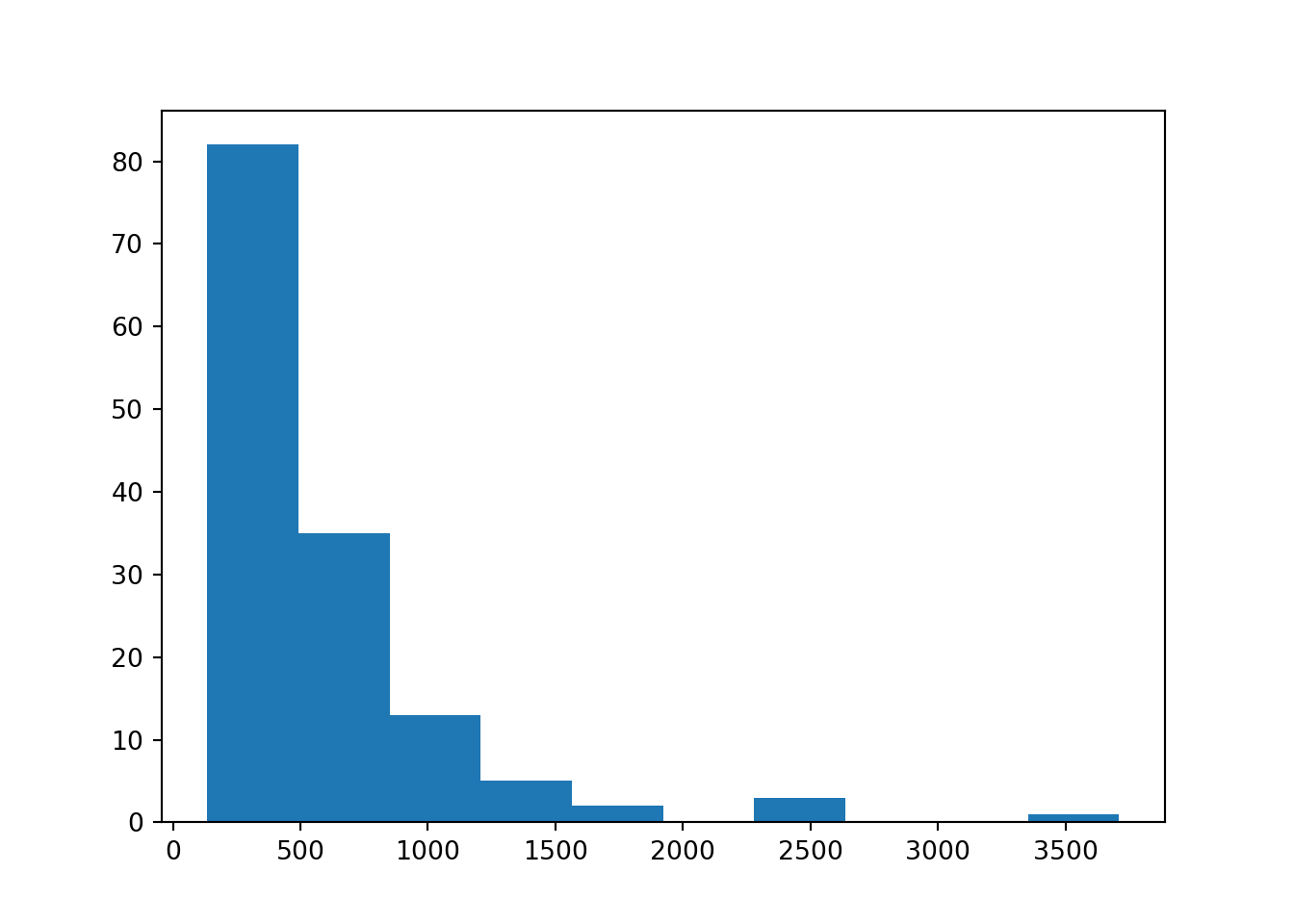### 2.1 Changing color

• To change the color of the plot,
``plt.hist(rivers['length'], color='darkorange')``
``plt.show()``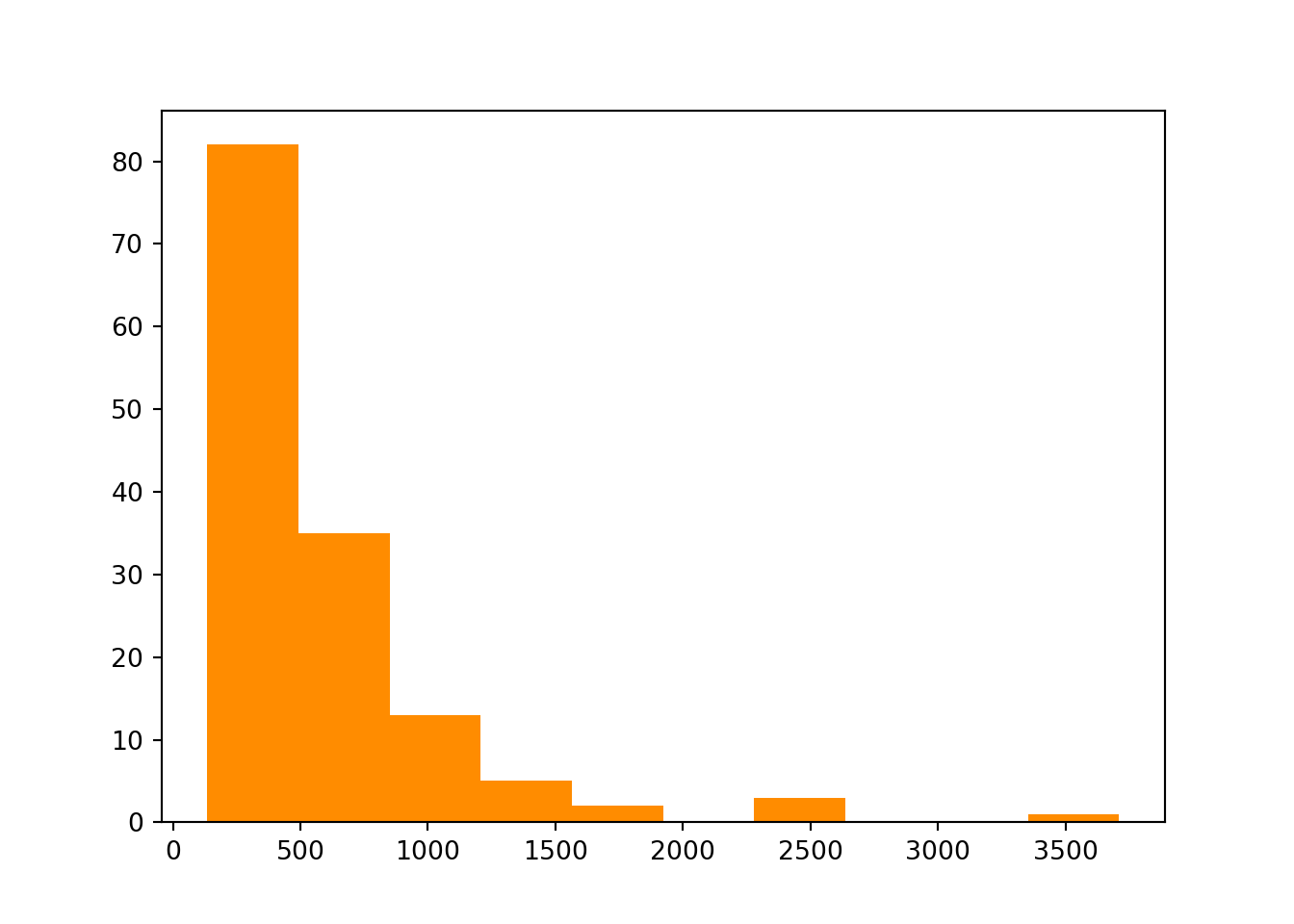• So what are the colors available to choose?

• Plots do not make sense without axis labels!
• To add an x-axis label in Python, use `plt.xlable()`:
``plt.hist(rivers['length'], color='darkorange')``
``````plt.xlabel('Length of river (in miles)')
plt.show()``````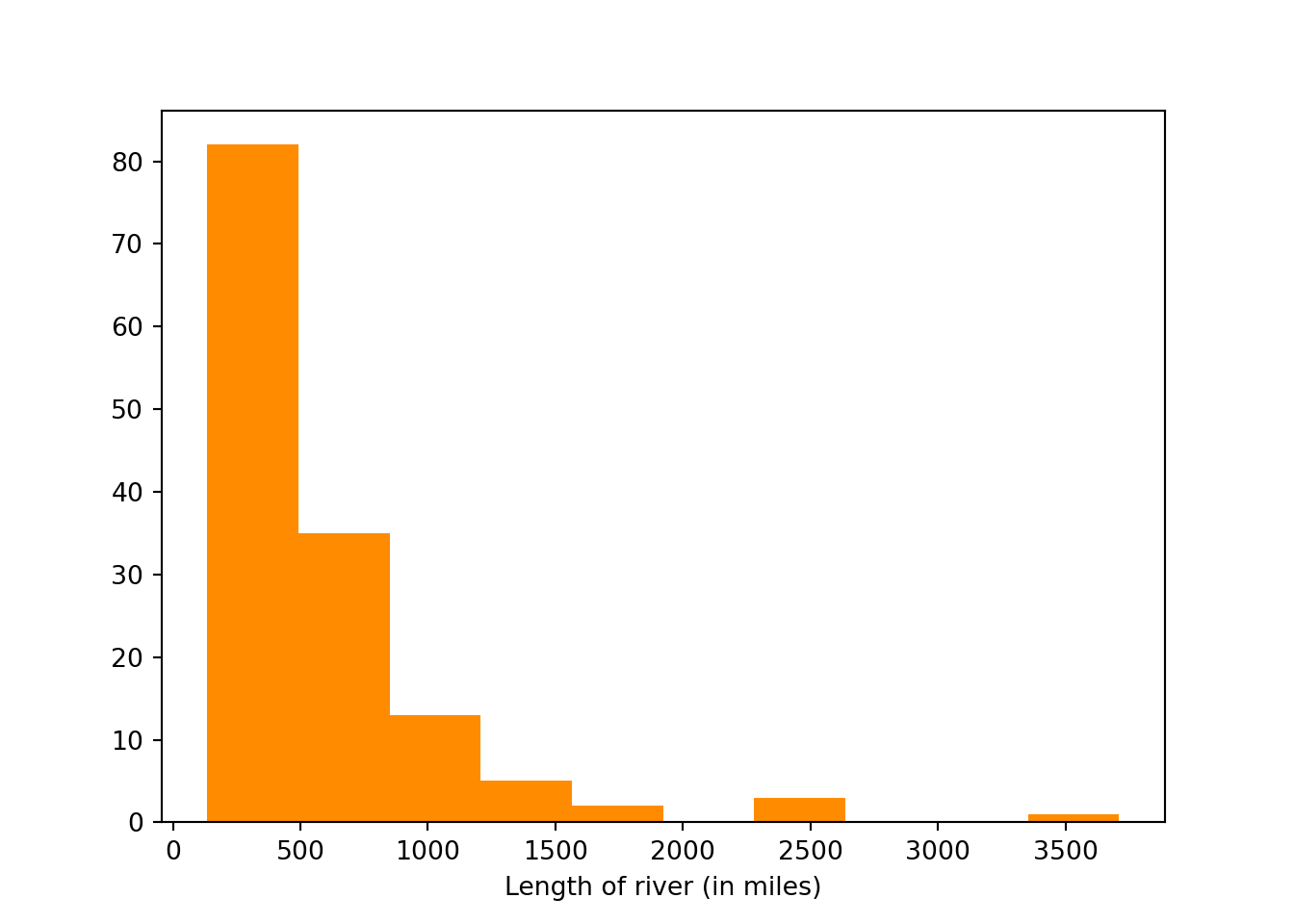• Similarly, use `plt.ylabel()` to add a y-axis label:
``plt.hist(rivers['length'], color='darkorange')``
``````plt.xlabel('Length of river (in miles)')
plt.ylabel('Count')
plt.show()``````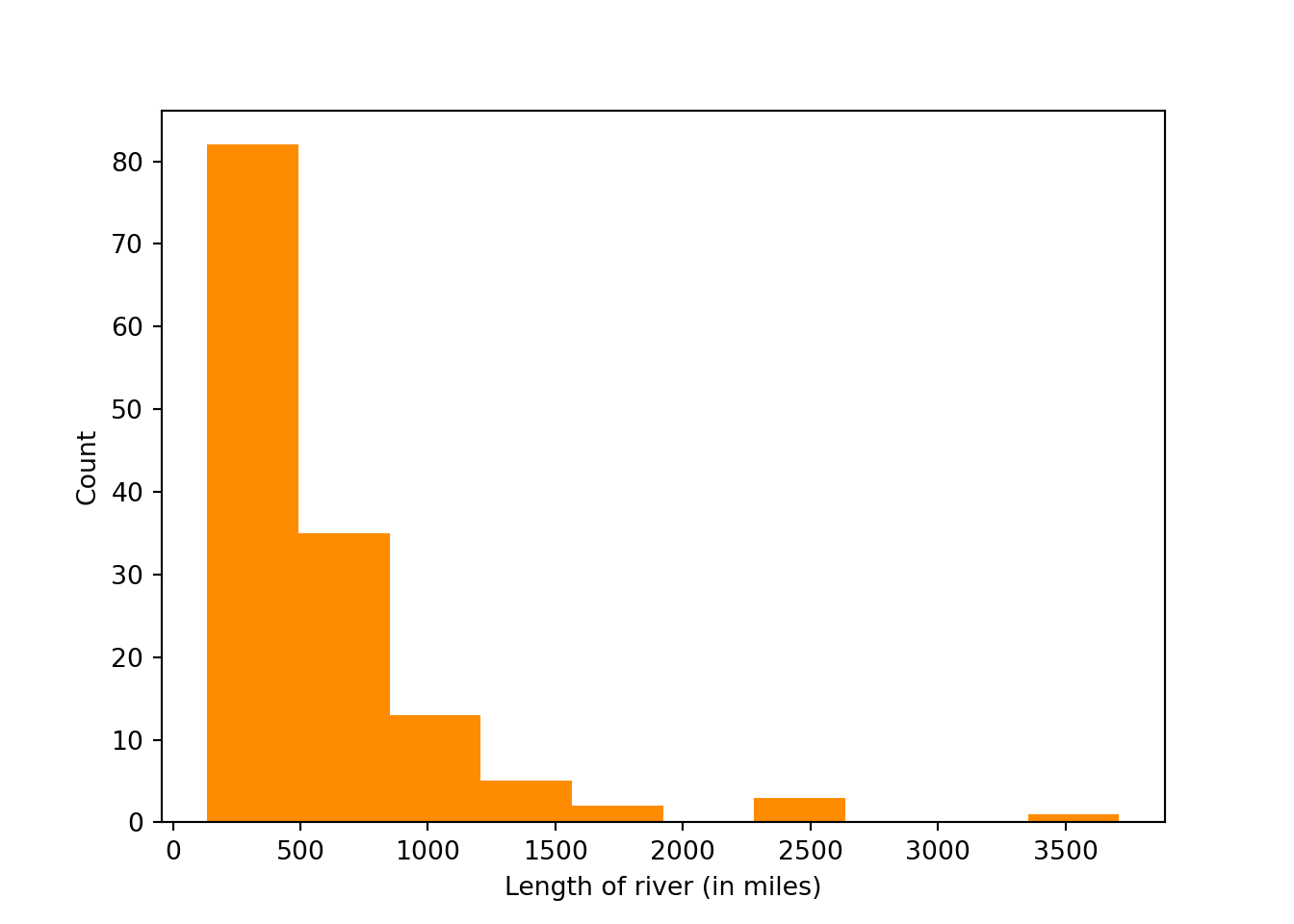### 2.3 Changing number of bins

• Histogram can look very different depending on the number of bins used to plot the histogram!
``plt.hist(rivers['length'], color='darkorange', bins=50)``
``plt.show()``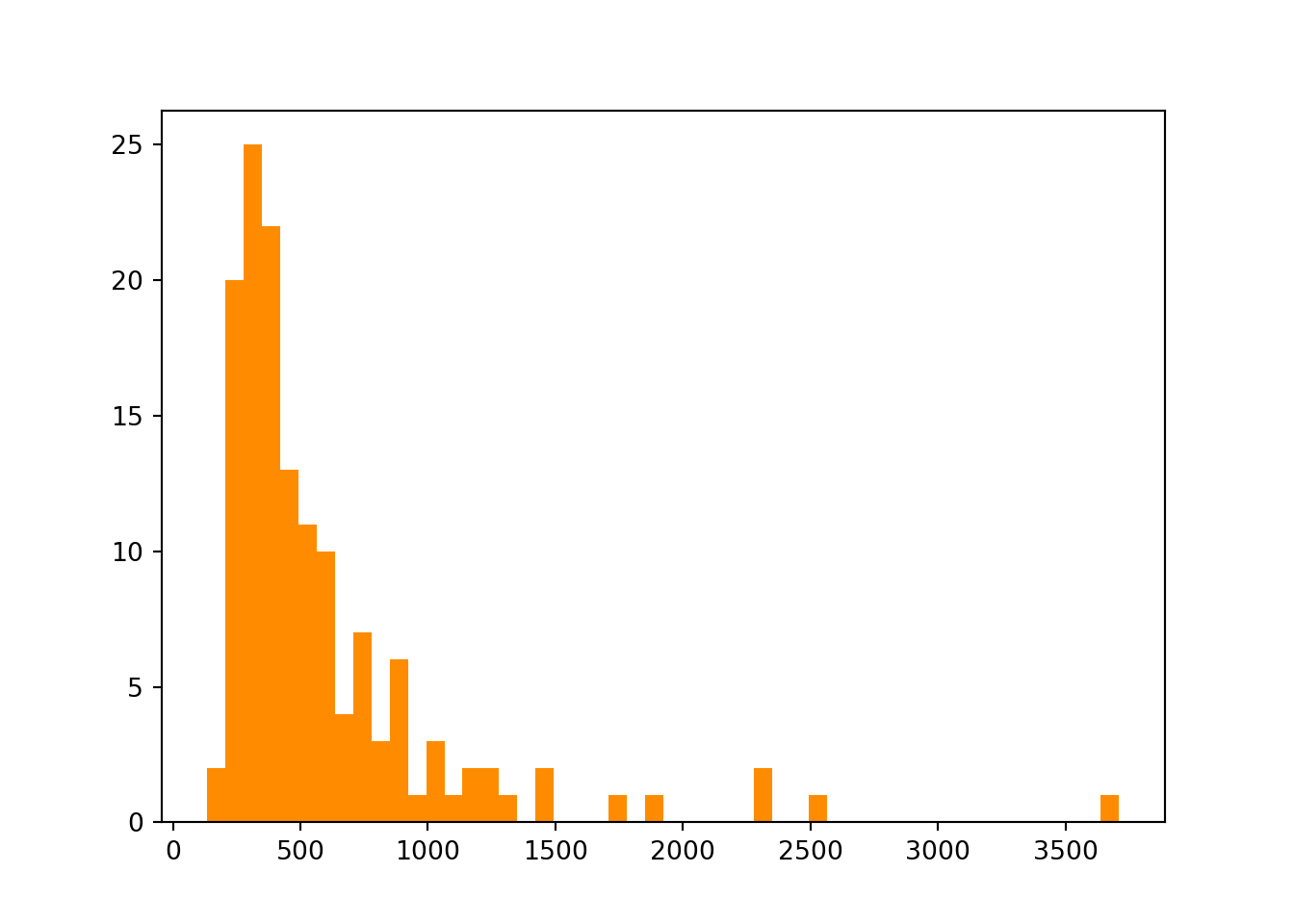``plt.hist(rivers['length'], color='darkorange', bins=5)``
``plt.show()``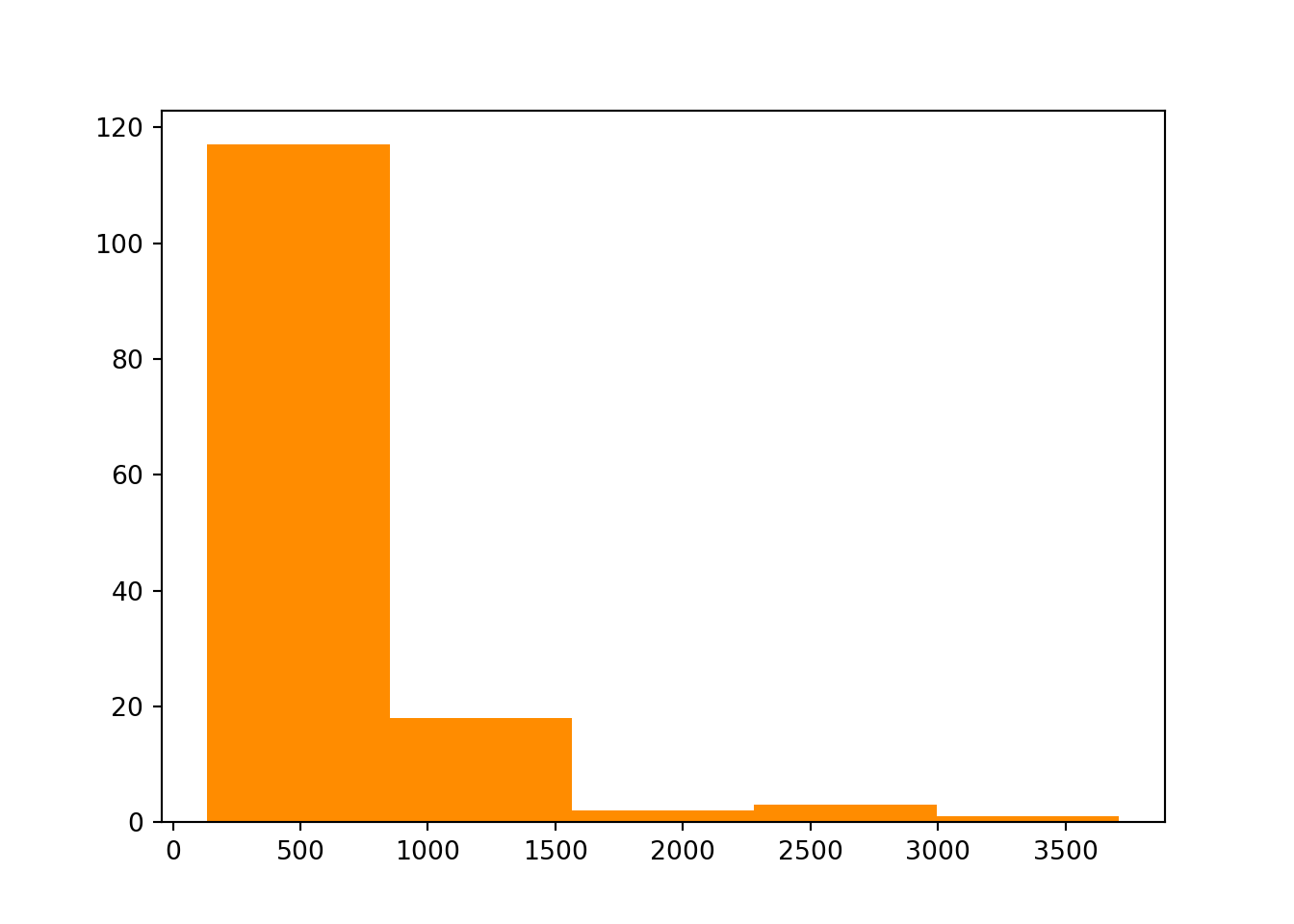## 3. Boxplot

• Use `plt.boxplot()` to plot a boxplot:
``plt.boxplot(rivers['length'])``
``plt.show()``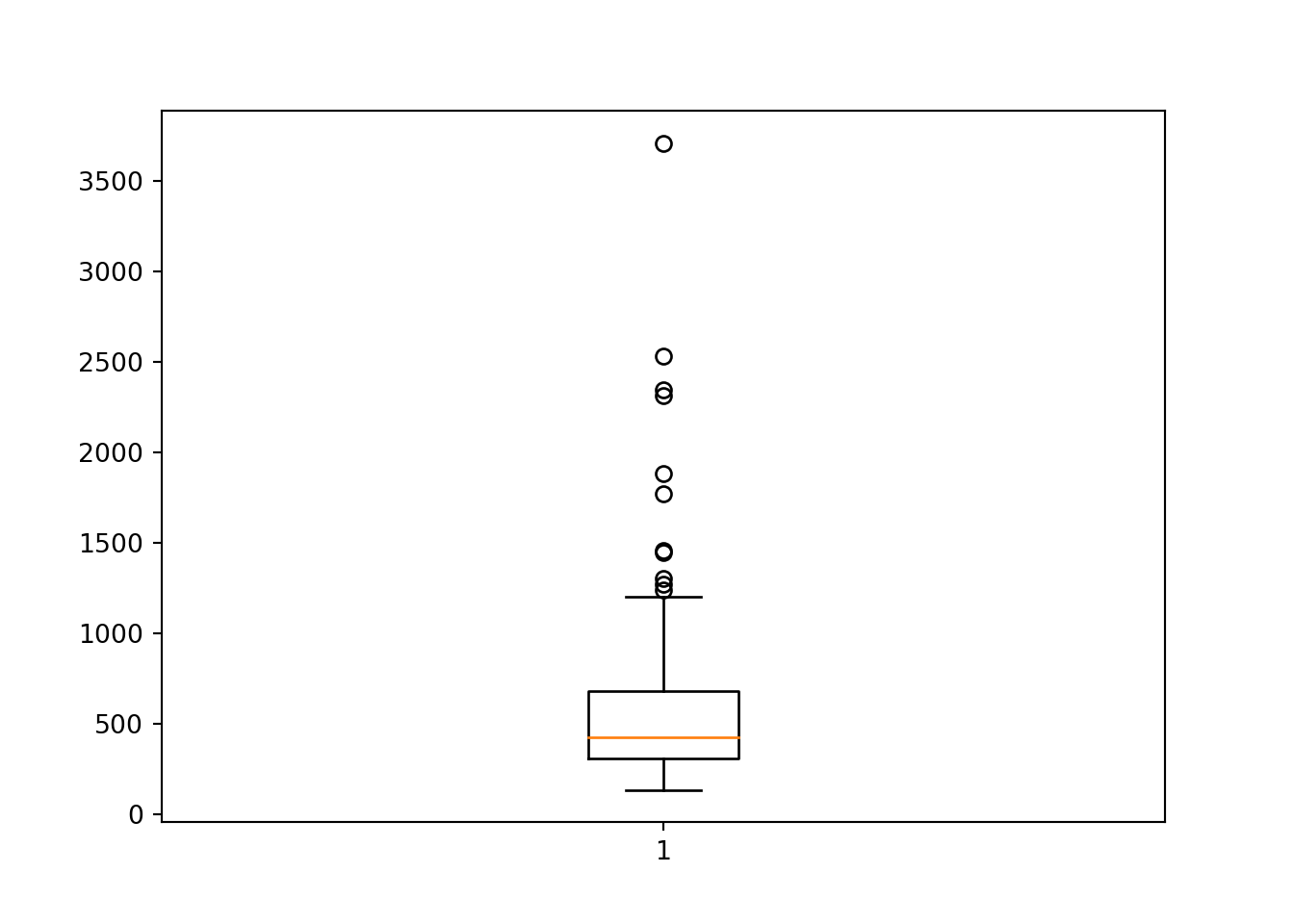### 3.1 Horizontal boxplot

• What’s if you want the boxplot to be horizontal instead?
``plt.boxplot(rivers['length'], vert=False)``
``plt.show()``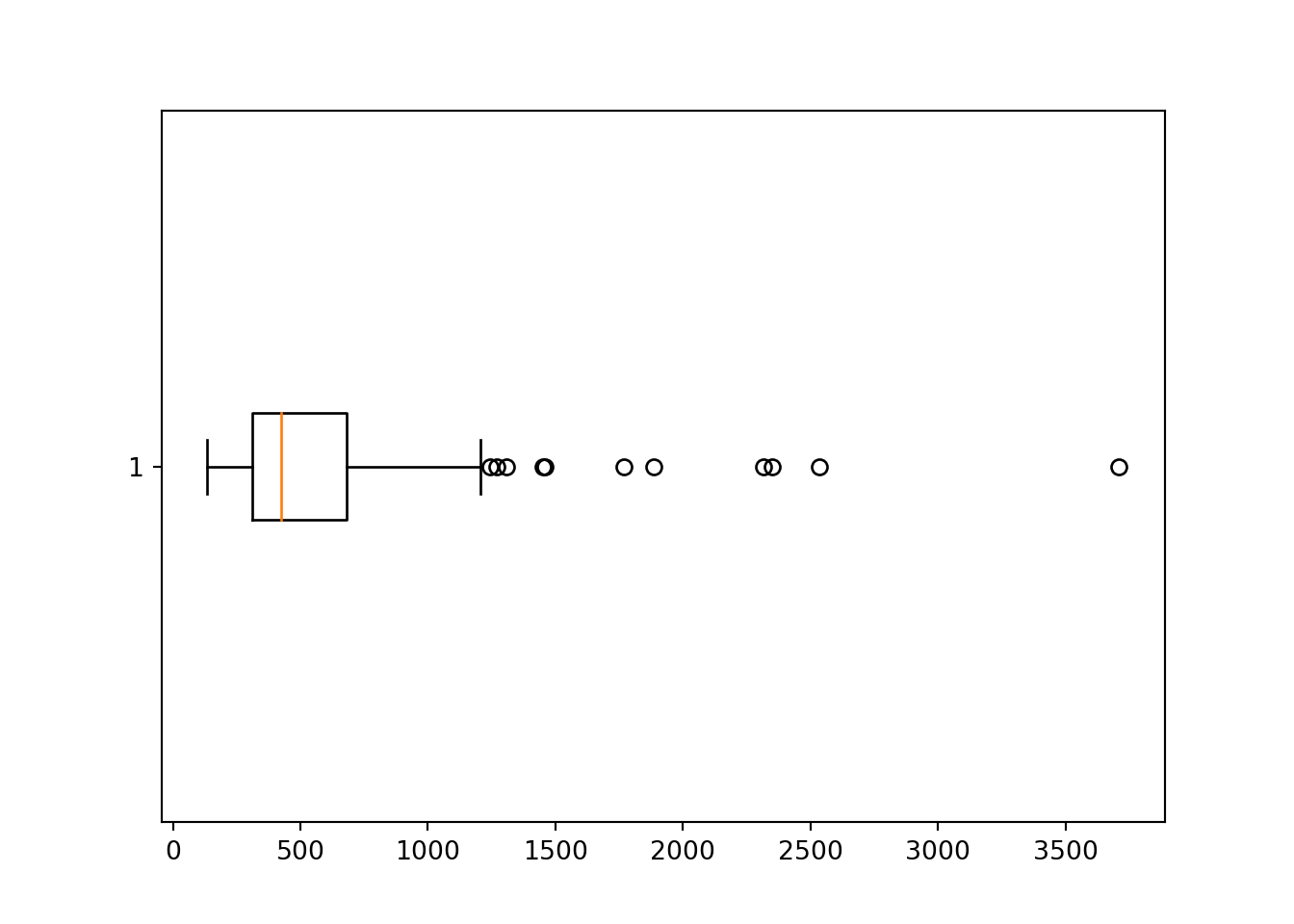### 3.2 Add variable name & axis label

• Just like with histogram, we can add axis label to boxplot! It might also be a good idea to add variable name (also called label) to the boxplot.
``plt.boxplot(rivers['length'], labels=['length'])``
``````plt.ylabel("Length of river (in miles)")
plt.show()``````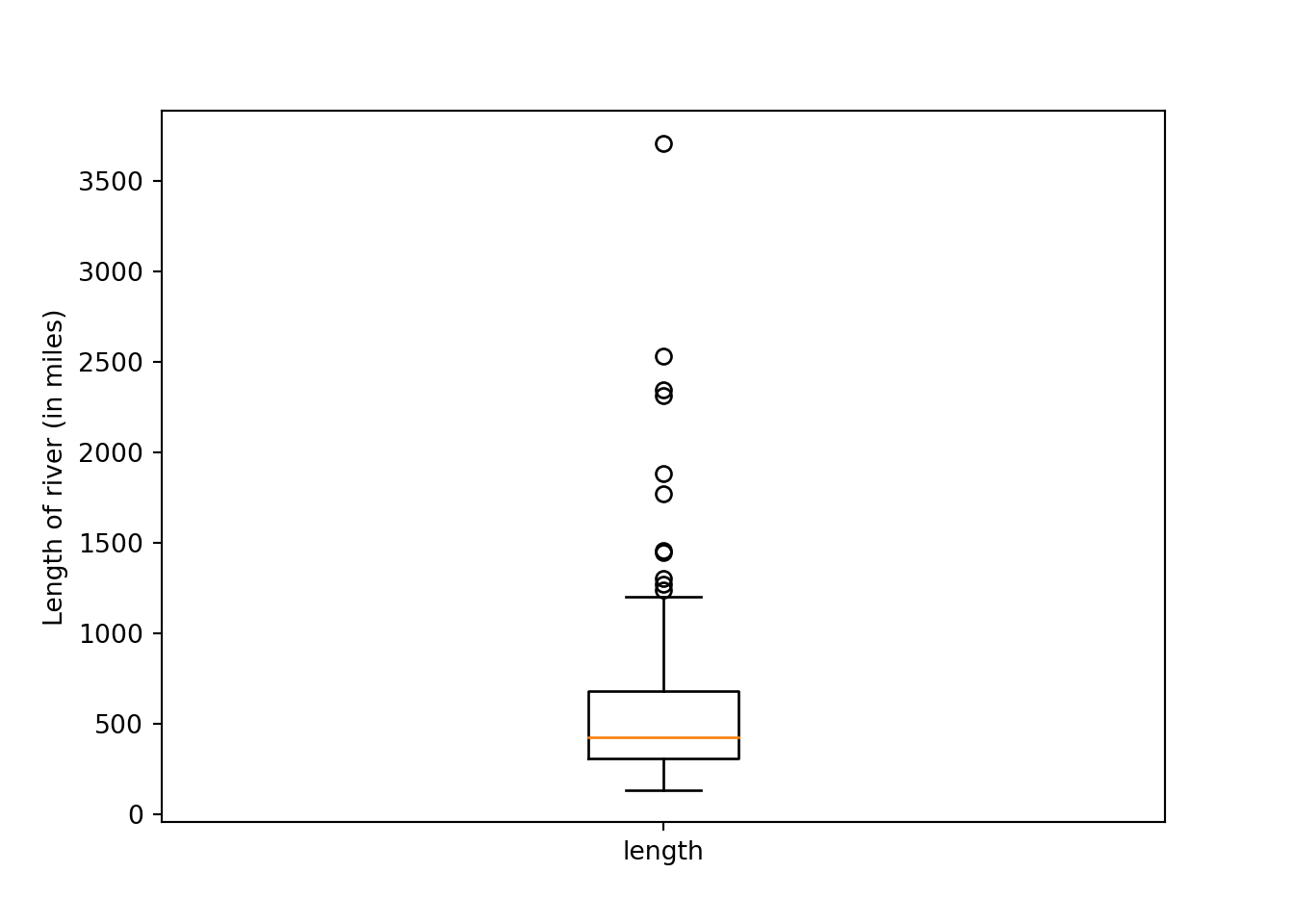• Adding variable names is especially important when you’re plotting multiple variables in the same plot!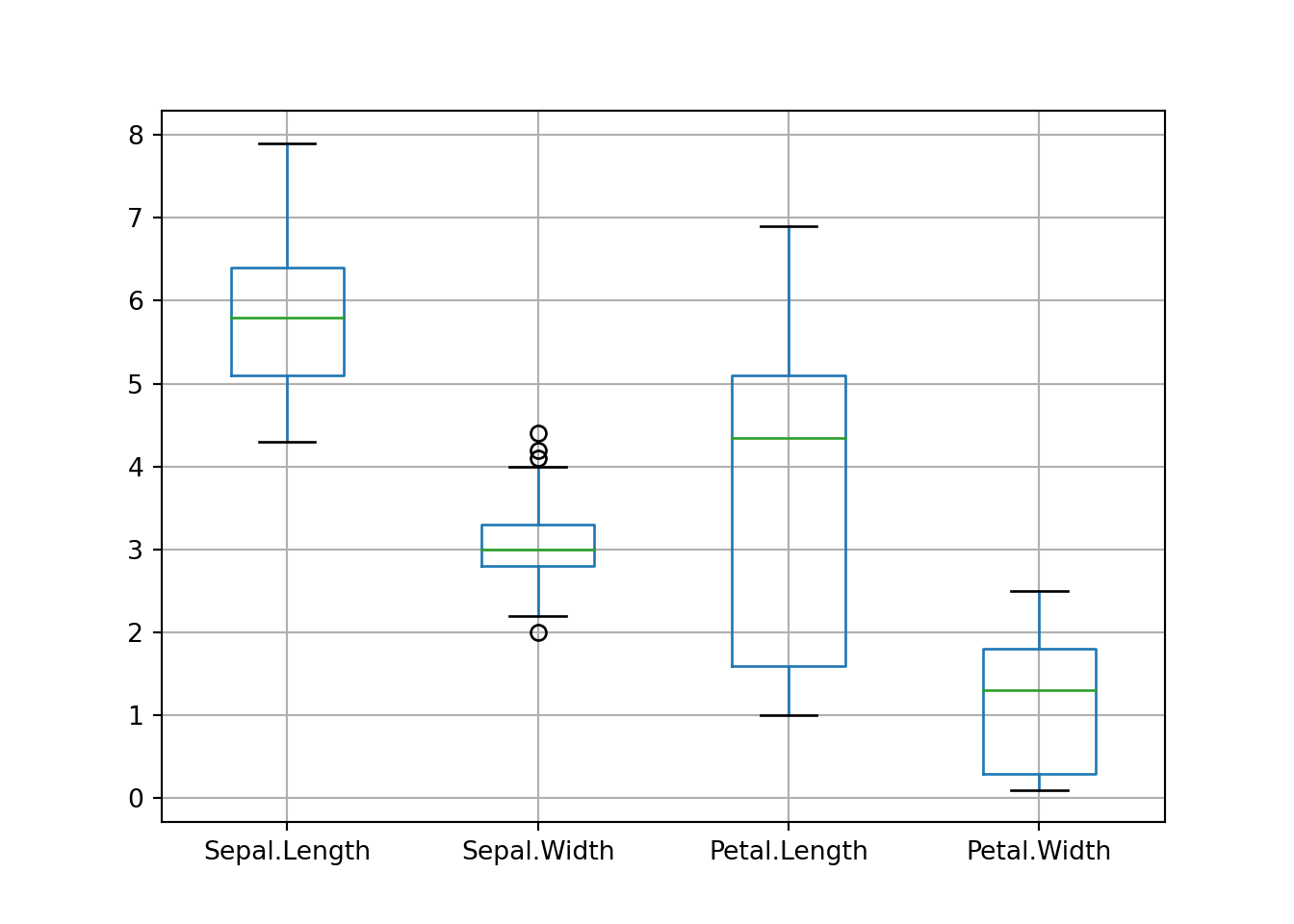## 4. Scatterplot

• Scatterplot is one of the most important plots in Statistics! We plot scatterplot in `Matplotlib` using `plt.scatter()`:
``plt.scatter(x=iris['Sepal.Length'], y=iris['Sepal.Width'])``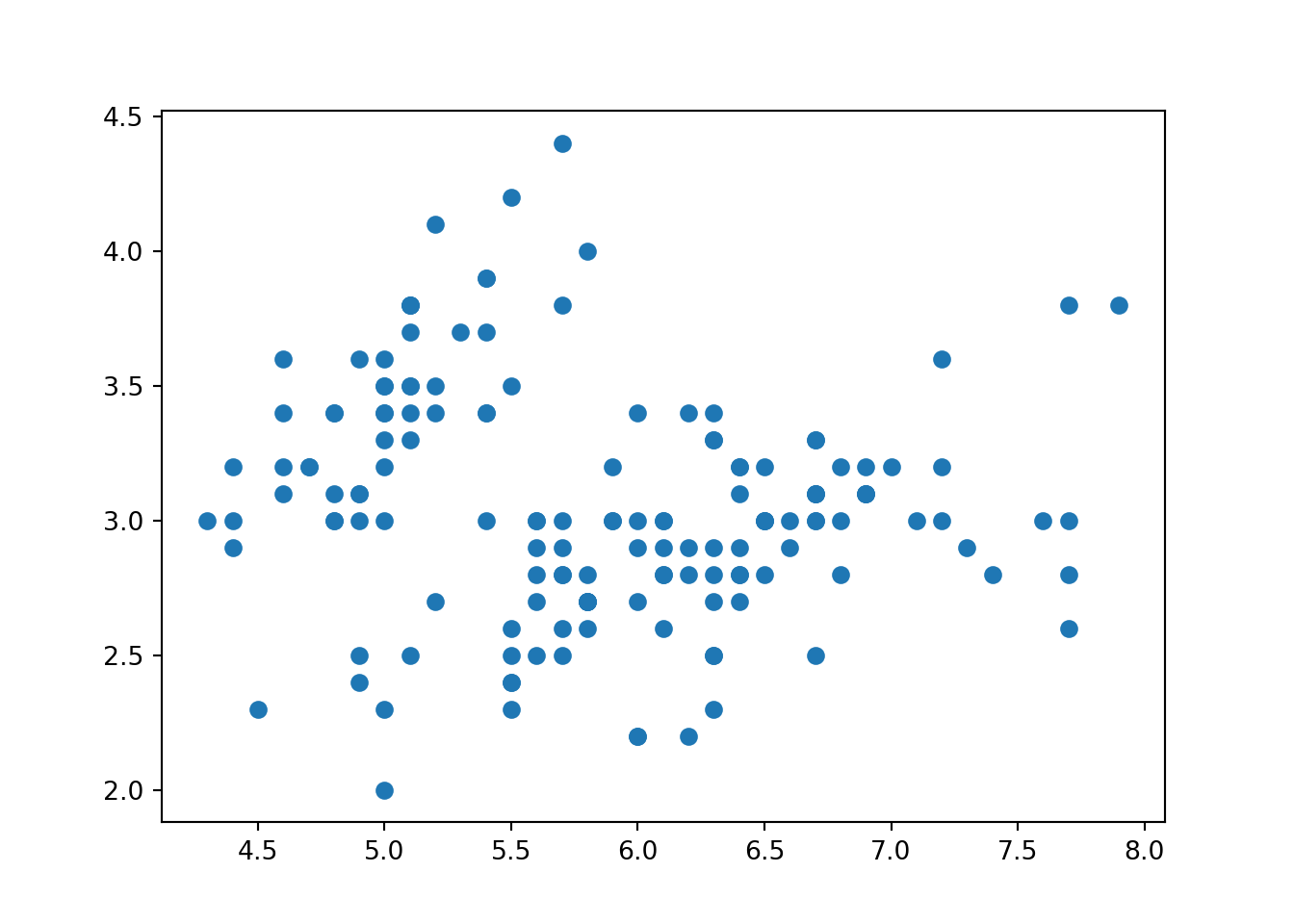• Now, let’s add axis labels and change the colors!
``````plt.scatter(x=iris['Sepal.Length'], y=iris['Sepal.Width'], color='darkorange')
plt.xlabel('Sepal Length (in cm)')
plt.ylabel('Sepal Width (in cm)')
plt.title('Iris Sepal Length vs. Sepal Width')``````